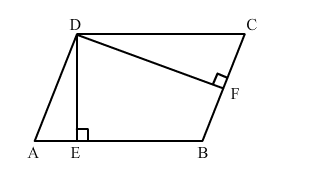# The adjacent sides of a parallelogram are 36 cm and 27 cm in length.`
Question:

The adjacent sides of a parallelogram are 36 cm and 27 cm in length. If the distance between the shorter sides is 12 cm, find the distance between the longer sides.

Solution:Area of a parallelogram $=$ Base $\times$ Height

$\therefore A B \times D E=B C \times D F$

$\Rightarrow D E=\frac{B C \times D F}{A B}$

$=\frac{27 \times 12}{36}$

$=9 \mathrm{~cm}$

∴ Distance between the longer sides = 9 cm